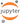# Pseudo Facebook Data - Plots in Python

In this post, we will learn about EDA of single variables using simple plots like histograms, frequency plots and box plots.

Data sets used below are part of a project from the UD651 course on udacity by Facebook. The data from the project corresponds to a typical data set at Facebook. You can load the data through the following command. Notice that this is a <TAB> delimited csv file. This data set consists of 99000 rows of data. We will see the details of different columns using the command below.

In :
import pandas as pd
import numpy as np
#Read csv file
pf = pd.readcsv("https://s3.amazonaws.com/udacity-hosted-downloads/ud651/pseudofacebook.tsv", sep = '\t')
#summarize data
pf.describe(include='all', percentiles=[]).T.replace(np.nan,' ', regex=True)

/p/ret/rettools/AnacondaPython/Python35/lib/python3.5/site-packages/numpy/lib/function_base.py:3403: RuntimeWarning: Invalid value encountered in median
RuntimeWarning)

Out:
count unique top freq mean std min 50% max
userid 99003.0 1.59705e+06 344059 1.00001e+06 1.59615e+06 2.19354e+06
age 99003.0 37.2802 22.5897 13 28 113
dob_day 99003.0 14.5304 9.01561 1 14 31
dob_year 99003.0 1975.72 22.5897 1900 1985 2000
dob_month 99003.0 6.28337 3.52967 1 6 12
gender 98828.0 2 male 58574
tenure 99001.0 537.887 457.65 0 3139
friend_count 99003.0 196.351 387.304 0 82 4923
friendships_initiated 99003.0 107.452 188.787 0 46 4144
likes 99003.0 156.079 572.281 0 11 25111
likes_received 99003.0 142.689 1387.92 0 8 261197
mobile_likes 99003.0 106.116 445.253 0 4 25111
mobile_likes_received 99003.0 84.1205 839.889 0 4 138561
www_likes 99003.0 49.9624 285.56 0 0 14865
www_likes_received 99003.0 58.5688 601.416 0 2 129953

We need convert some of the variables from numeric to category.

In :
cats = ['userid', 'dob_day', 'dob_year', 'dob_month']
for col in pf.columns:
if col in cats:
pf[col] = pf[col].astype('category')
#summarize data
pf.describe(include='all', percentiles=[]).T.replace(np.nan,' ', regex=True)

/p/ret/rettools/AnacondaPython/Python35/lib/python3.5/site-packages/numpy/lib/function_base.py:3403: RuntimeWarning: Invalid value encountered in median
RuntimeWarning)

Out:
count unique top freq mean std min 50% max
userid 99003.0 99003 2.19354e+06 1
age 99003.0 37.2802 22.5897 13 28 113
dob_day 99003.0 31 1 7900
dob_year 99003.0 101 1995 5196
dob_month 99003.0 12 1 11772
gender 98828.0 2 male 58574
tenure 99001.0 537.887 457.65 0 3139
friend_count 99003.0 196.351 387.304 0 82 4923
friendships_initiated 99003.0 107.452 188.787 0 46 4144
likes 99003.0 156.079 572.281 0 11 25111
likes_received 99003.0 142.689 1387.92 0 8 261197
mobile_likes 99003.0 106.116 445.253 0 4 25111
mobile_likes_received 99003.0 84.1205 839.889 0 4 138561
www_likes 99003.0 49.9624 285.56 0 0 14865
www_likes_received 99003.0 58.5688 601.416 0 2 129953

The goal of this analysis is to understand user behavior and their demographics. We want to understand what they are doing on the Facebook and what they use. Please note this is not a real Facebook dataset.

Our goal is to do some basic EDA (Exploratory Data Analysis) to understand any underlying patterns in the data. We will first look at a histogram of User's Birthdays.

In :
import matplotlib.pyplot as plt
import seaborn as sns
sns.set(style="darkgrid")
%matplotlib inline
ax = sns.countplot(x="dob_day", data=pf)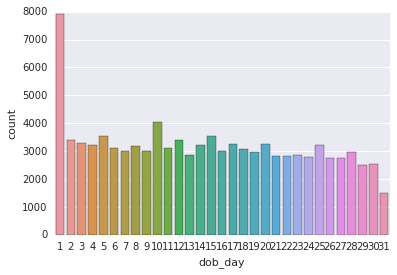We see some peculiar behavior of the data on the 1st of the month. Let us plot this data in more detail, in per month basis.

In :
g = sns.factorplot("dob_day", col="dob_month", col_wrap=4, data=pf, kind='count', size=2.5, aspect=.8)
g.set(xticklabels=[])

Out:
<seaborn.axisgrid.FacetGrid at 0x7fffcd441208>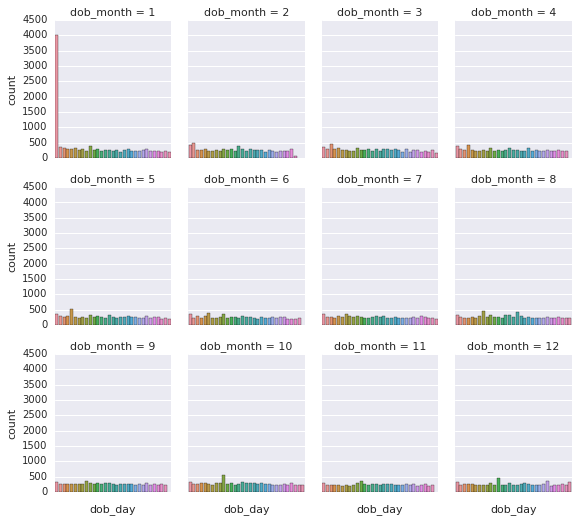This explains the above plot. Because of the default settings, or users privacy concerns, numerous people have 1/1 as their birthdays!

Now, let us explore the distribution of friend counts in this data.

In :
ax = sns.distplot(pf["friend_count"], kde=False, bins=100)
plt.xlim(0,1000)

Out:
(0, 1000)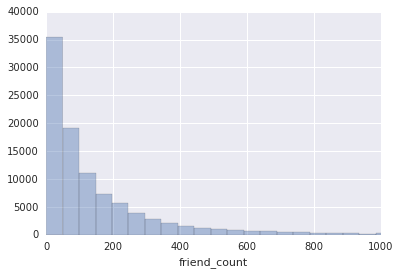We see the data has some outliers near 5000. This is an example of a long tail data. We want our analysis to be focused on the bunch of Facebook users, so we need to limit the axes of these plots. Additionally, we also want to look at these data as a function of gender. However, We also want to remove any data where gender is NA.

In :
df = pf[pf.gender.notnull()]
g = sns.FacetGrid(df, col="gender")
g = g.map(plt.hist, "friend_count", bins=100, color="b")
plt.xlim(0,1000)

Out:
(0, 1000)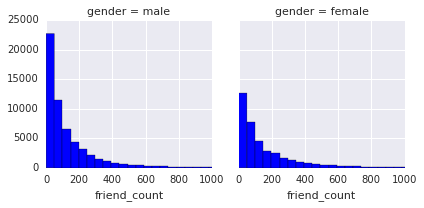If we want to know, mean statistics of our data, we can use the 'value_counts' command.

In :
pf.groupby('gender').friend_count.describe()

Out:
gender
female  count    40254.000000
mean       241.969941
std        476.039706
min          0.000000
25%         37.000000
50%         96.000000
75%        244.000000
max       4923.000000
male    count    58574.000000
mean       165.035459
std        308.466702
min          0.000000
25%         27.000000
50%         74.000000
75%        182.000000
max       4917.000000
Name: friend_count, dtype: float64

Let us know look at the tenure of usage (measured in Years) of Facebook.

In :
df = pf[pf.tenure.notnull()]
ax = sns.distplot(df["tenure"]/365, kde=False, bins=36)
plt.xlim(0,7)
plt.xlabel('Number of years using Facebook', fontsize=12)
plt.ylabel('Number of users in sample', fontsize=12)

Out:
<matplotlib.text.Text at 0x7fffc9590ef0>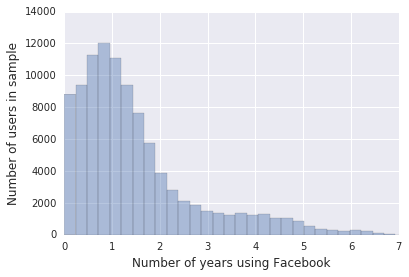We will now look at any pattern in the ages of Facebook users in this dataset.

In :
ax = sns.distplot(pf["age"], kde=False, bins=100)
plt.xlim(13,113)
plt.xlabel('Age of Users in Years', fontsize=12)
plt.ylabel('Number of users in sample', fontsize=12)

Out:
<matplotlib.text.Text at 0x7fffc94f7780>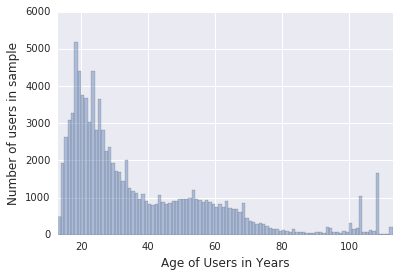One general theme of observation here is that most of the data have a long tail. In these circumstances, it is better to look at such data after certain types of transformation. Let us do such an analysis of “friend_count”.

In :
ax = sns.distplot(pf["friend_count"], kde=False, hist_kws={"alpha": 0.9})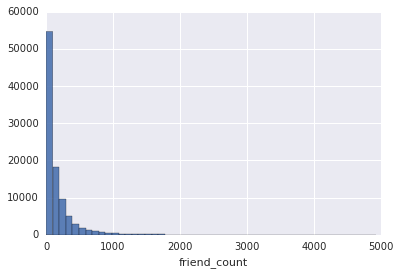In :
ax = sns.distplot(pf["friend_count"], kde=False, bins=np.logspace(0,4), hist_kws={"alpha": 0.9})
plt.xscale('log')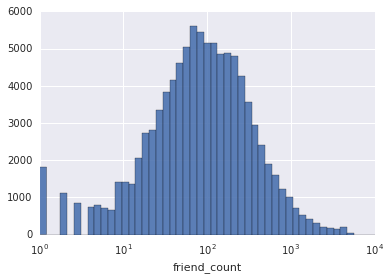Let us try to compare distribution of male vs female friend counts.

In :
def plotDensity(x, color=None, label=None, bins=np.linspace(0,1000,200), **kws):

w = 100*np.ones_like(x)/x.size
plt.hist(x, bins=bins, alpha=0.4, histtype='step', linewidth=2, label=label, color=color, weights=w, **kws)
return

g = sns.FacetGrid(df, col=None, hue='gender', size=6.0, xlim=(6,600), ylim=(0,5), legendout=True)
g = (g.map(plotDensity, 'friendcount')).addlegend()
g = g.setaxis_labels('Friend Count', '% of users')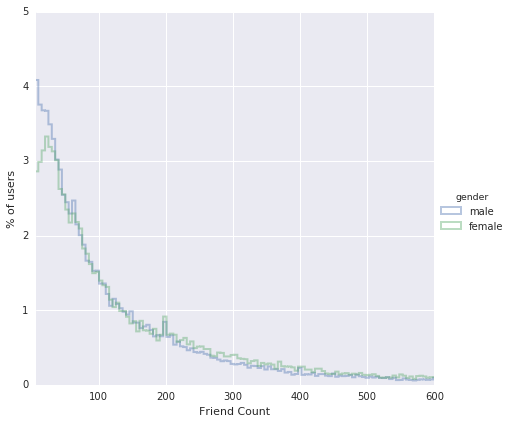Similarly, we can compare distributions of www likes.

In :
g = sns.FacetGrid(df, col=None, hue='gender', size=6.0, xlim=(1,15000))
g = (g.map(plotDensity, 'www_likes', bins=np.logspace(0,5,50))).add_legend()
g = g.set_axis_labels('www Likes Count', '% of users')
plt.xscale('log')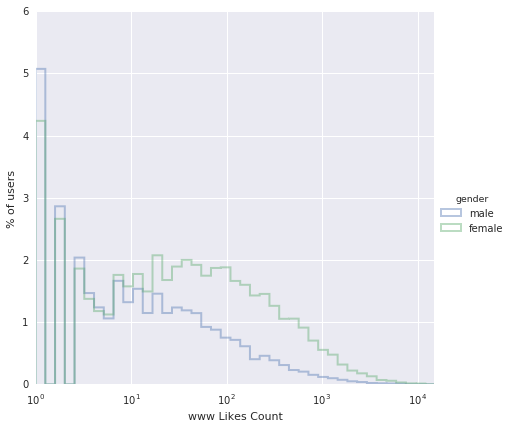We cal also look at the total number of likes numerically per gender, as follows:

In :
pf.groupby('gender').www_likes.sum()

Out:
gender
female    3507665
male      1430175
Name: www_likes, dtype: int64

We can also compare two distributions graphically using “box plots”. We can also look at the actual value using the by command. Here, we are trying to understand which gender initiated more friendships.

In :
ax = sns.boxplot(x='gender', y='friendships_initiated', data=df)
plt.ylim(0,200)

Out:
(0, 200)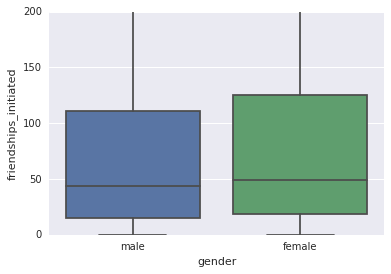In :
pf.groupby('gender').friendships_initiated.describe()

Out:
gender
female  count    40254.000000
mean       113.899091
std        195.139308
min          0.000000
25%         19.000000
50%         49.000000
75%        124.750000
max       3654.000000
male    count    58574.000000
mean       103.066600
std        184.292570
min          0.000000
25%         15.000000
50%         44.000000
75%        111.000000
max       4144.000000
Name: friendships_initiated, dtype: float64

Next, we want to understand if users have used certain features of Facebook or not. If we look at the summary of mobile_likes variable, median is close to 0, indicating a lot many users with 0 values for this variable. We can look also look at the logical value if value of this quantity is non-zero. We can additionally create a new variable called mobile_check_in that takes a value 1 if mobile_likes is non-zero.

In :
pf.mobile_likes.describe()

Out:
count    99003.000000
mean       106.116300
std        445.252985
min          0.000000
25%          0.000000
50%          4.000000
75%         46.000000
max      25111.000000
Name: mobile_likes, dtype: float64
In :
(pf.mobile_likes > 0).value_counts()

Out:
True     63947
False    35056
Name: mobile_likes, dtype: int64
In :
pf['mobile_check_in'] = pd.Series(np.where(pf['mobile_likes'] > 0, 1, 0)).astype('category')
pf.mobile_check_in.value_counts()

Out:
1    63947
0    35056
Name: mobile_check_in, dtype: int64

We can find percentage of people who have done mobile check in.

In :
frac = (pf.mobile_check_in == 1).sum()/pf.mobile_check_in.size
print("Fraction of Mobile Check-ins = ", frac)

Fraction of Mobile Check-ins =  0.645909719907


We find that about 65% of people have used mobile devices for check in and hence it would be a good decision to continue development of such products.

In summary, here we have learned to make inferences about single variable data using a combination of plots - histograms, box plots and frequency plots; along with various numerical data.

Share This:

## Comments

### Stay in touch

Like the posts you see here? Sign up to get notified about new ones.

# Get The Best Of Posts Delivered To Your Inbox

Subscribe to my newsletter and stay updated.